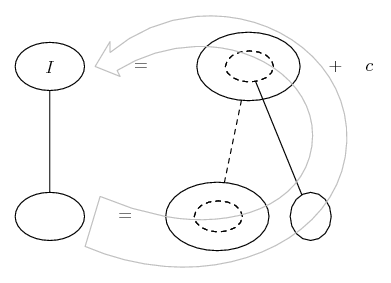# Thread: Integate with arcsin x^1/2

1. ## Integate with arcsin x^1/2

I need to integrate with direct integration method
$\displaystyle \int\frac{\arcsin \sqrt x}{\sqrt{x - x^2}}$

I do get $\displaystyle d(x-x^2)=1-2x d(x-x^2)$ then $\displaystyle \int\frac{\arcsin \sqrt x}{(x - x^2)^\frac{1}{2}}\frac{1}{1-2x}\d(x-x^2)=$ and that where my brains stop, I don't know what to to with that $\displaystyle \arcsin \sqrt x$

2.Originally Posted by RevyI need to integrate with direct integration method
$\displaystyle \int\frac{\arcsin \sqrt x}{\sqrt{x - x^2}}$

I do get $\displaystyle \d(x-x^2)=1-2x\d(x-x^2)$ then $\displaystyle \int\frac{\arcsin \sqrt x}{(x - x^2)^\frac{1}{2}}\frac{1}{1-2x}\d(x-x^2)=$ and that where my brains stop, I don't know what to to with that $\displaystyle \arcsin \sqrt x$
Try making the substitution $\displaystyle u = \arcsin\sqrt x$. Then $\displaystyle \frac{du}{dx} = \frac1{2\sqrt{1-x}\sqrt x} = \frac1{2\sqrt{x-x^2}}$ and the integral becomes $\displaystyle \displaystyle\int\!\!2u\,du = u^2 = \bigl(\arcsin\sqrt x\bigr)^2$ (plus a constant of integration).

3. For this task I can't use substitution, have to do it directly. But thanks, this one will be useful as well

Just one thing, how do you get denominator in
$\displaystyle \frac{du}{dx} = \frac1{2\sqrt{1-x}\sqrt x} = \frac1{2\sqrt{x-x^2}}$?

4.Originally Posted by RevyJust one thing, how do you get denominator in
$\displaystyle \frac{du}{dx} = \frac1{2\sqrt{1-x}\sqrt x} = \frac1{2\sqrt{x-x^2}}$?
$\displaystyle \sqrt{a} \cdot \sqrt{b} = \sqrt{a \cdot b}$

5. Sorry, I should have asked more accurately.
I wanted to know why there is $\displaystyle 2$ and not $\displaystyle 1-2x$ from $\displaystyle d(x-x^2)=1-2x d(x-x^2)$
Do you differentiate $\displaystyle 1-2x$ part? I don't understand the meaning of $\displaystyle \frac{du}{dx}$

Since we know formula $\displaystyle \arcsin{x} = \frac1{\sqrt{1-x^2}}$ how can you get $\displaystyle \arcsin{\sqrt{x}}$ from $\displaystyle \frac1{\sqrt{x-x^2}}$?

Shouldn't in this case equality be $\displaystyle \arcsin{\sqrt{x}} = \frac1{\sqrt{1-x}}$? If that's the case, then where does $\displaystyle \sqrt x$ disappear from $\displaystyle \frac1{2\sqrt{1-x}\sqrt x}$ and how $\displaystyle 2$ gets from denominator to numerator?

Sorry, I must be a headache.

6. Just in case a picture helps...

Differentiate arcsin root x (travelling downwards)...... where (key in spoiler) ...

Spoiler:... is the chain rule. Straight continuous lines differentiate downwards (integrate up) with respect to the main variable (in this case ), and the straight dashed line similarly but with respect to the dashed balloon expression (the inner function of the composite which is subject to the chain rule).

Then, working backwards through the chain rule to integrate the whole of the original fraction...... where (key in spoiler) ...

Spoiler:

The general drift is...Spoiler:Use the same diagram to check the integration by differentiating your result (i.e. by working down/clockwise instead of up/anti-clockwise through the same picture).
_________________________________________

Don't integrate - balloontegrate!

Balloon Calculus; standard integrals, derivatives and methods

Balloon Calculus Drawing with LaTeX and Asymptote!

7. Thanks, it was hard, but seems I did understood it

8.Originally Posted by RevyThanks, it was hard, but seems I did understood it
General rule of integration is

$\displaystyle \int{f(x)f'(x)}dx = [f(x)]^2$. Here f'(x) is the derivative of f(x)

In the given problem f(x) = arcsin(x) . Find f'(x)

,

,

,

,

,

,

,

,

### find intigration 1/x^1/2 x^1/3

Click on a term to search for related topics.